# Types of Angles | Learn with Real Life Examples

1. Math Lessons >
2. Types of Angles

Based on their measurements, angles are classified into different types as follows.

Acute angle: Less than 90º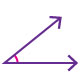Right angle: Equal to 90º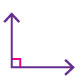Obtuse angle: More than 90º but less than 180º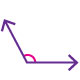Straight angle: Equal to 180º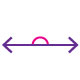Reflex angle: More than 180º but less than 360º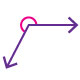Complete angle: Equal to 360º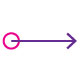Examples of different angles inspired from real life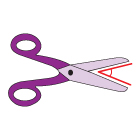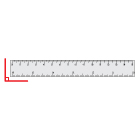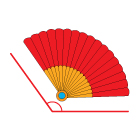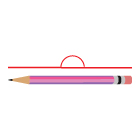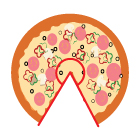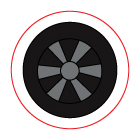Bringing all types of angles together!

Observe the range of each type of angle and comprehend the classification instantly.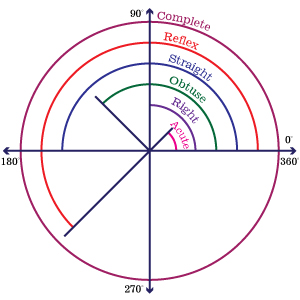Identifying types of angles

Hover on each image to identify its type.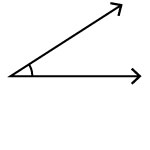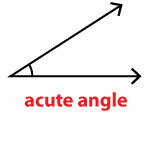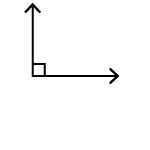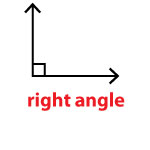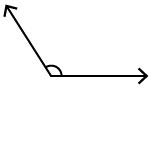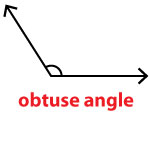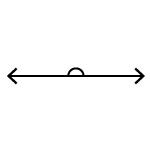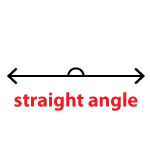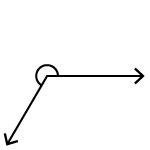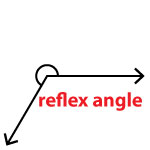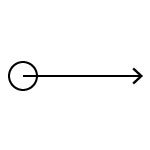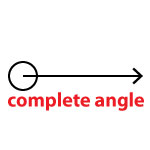Have fun with angles!

Count the number of acute and right angles in each letter of the word 'MATH'.

Hover on each letter to see the position of the angles.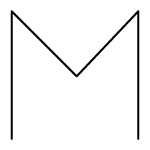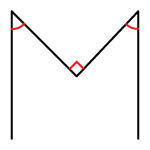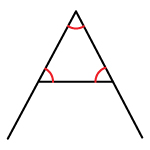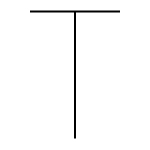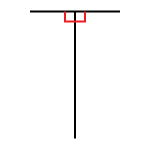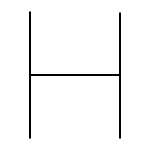Did you count 12? Math is a real game changer, isn't it?Feed your skills with lots of informed practice, using our free printable Types of Angles worksheets.